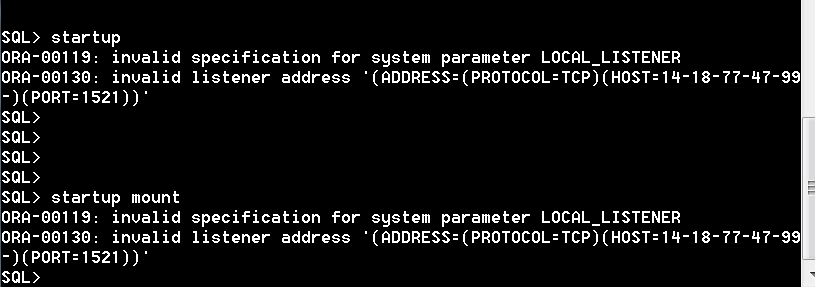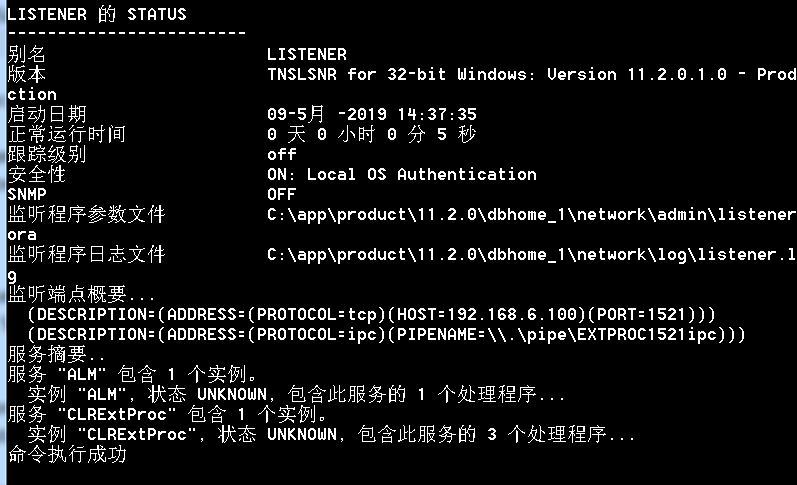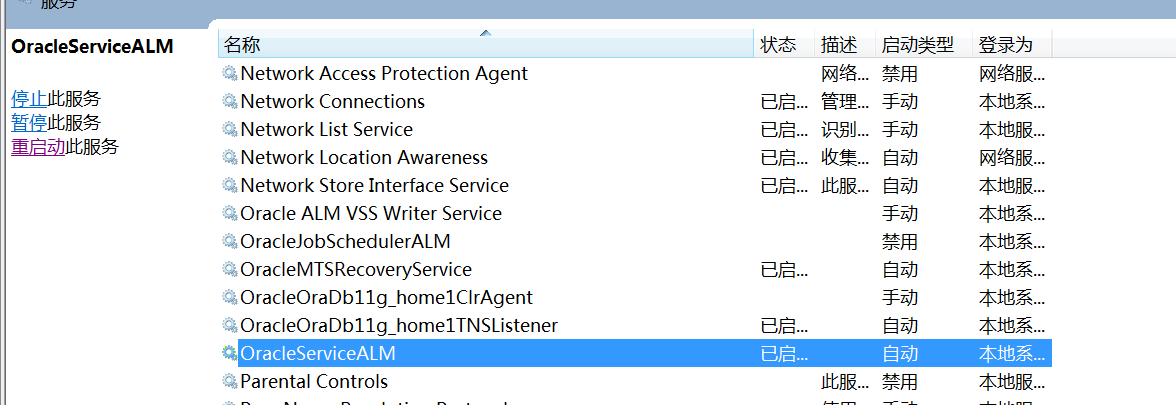##### Windows平台Oracle 启动数据失败：ora-00119和ora-00130，如何解决？# listener.ora Network Configuration File: C:\app\product\11.2.0\dbhome_1\network\admin\listener.ora
# Generated by Oracle configuration tools.

SID_LIST_LISTENER =
(SID_LIST =
(SID_DESC =
(SID_NAME = CLRExtProc)
(ORACLE_HOME = C:\app\product\11.2.0\dbhome_1)
(PROGRAM = extproc)
(ENVS = "EXTPROC_DLLS=ONLY:C:\app\product\11.2.0\dbhome_1\bin\oraclr11.dll")
)
(SID_DESC =
(GLOBAL_DBNAME = ALM)
(SID_NAME = ALM)
)
(SID_DESC =
(SID_NAME = CLRExtProc)
(ORACLE_HOME = C:\app\product\11.2.0\dbhome_1)
(PROGRAM = extproc)
(ENVS = "EXTPROC_DLLS=ONLY:C:\app\product\11.2.0\dbhome_1\bin\oraclr11.dll")
)
(SID_DESC =
(SID_NAME = CLRExtProc)
(ORACLE_HOME = C:\app\product\11.2.0\dbhome_1)
(PROGRAM = extproc)
(ENVS = "EXTPROC_DLLS=ONLY:C:\app\product\11.2.0\dbhome_1\bin\oraclr11.dll")
)
)

LISTENER =
(DESCRIPTION_LIST =
(DESCRIPTION =
(ADDRESS = (PROTOCOL = TCP)(HOST = 192.168.6.100)(PORT = 1521))
(ADDRESS = (PROTOCOL = IPC)(KEY = EXTPROC1521))
)
)

ADR_BASE_LISTENER = C:\app

# tnsnames.ora Network Configuration File: C:\app\product\11.2.0\dbhome_1\network\admin\tnsnames.ora
# Generated by Oracle configuration tools.

ORACLR_CONNECTION_DATA =
(DESCRIPTION =
(ADDRESS_LIST =
(ADDRESS = (PROTOCOL = IPC)(KEY = EXTPROC1521))
)
(CONNECT_DATA =
(SID = CLRExtProc)
(PRESENTATION = RO)
)
)

ALM =
(DESCRIPTION =
(ADDRESS = (PROTOCOL = TCP)(HOST = 192.168.6.100)(PORT = 1521))
(CONNECT_DATA =
(SERVER = ALM)
(SERVICE_NAME = ALM)
)
)
...全文
183 点赞 收藏 16

16 条回复6391

5.4w+

Oracle开发相关技术讨论